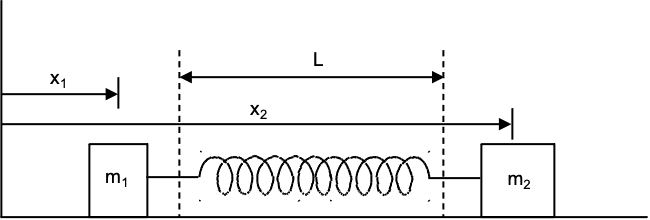# Derive angular frequency for mass spring system

so_gr_lo
Homework Statement:
Two masses m1 and m2 on the x axis are connected by a spring. The spring has stiffness s, length l and extension x. m2 is at position x2 and m1 at position x1. The equations of the motion are

m1d^2x1/dt^2 = sx and m2d^2x2/dt^2 = -sx

Combine these to show that the angular frequency is w = sqrt(s/M)

Where M = m1m2/ m1 + m2 (the reduced mass)
Relevant Equations:
m1d^2x1/dt^2 = sx and m2d^2x2/dt^2 = -sx

w = sqrt(s/M)

M = m1m2/ m1 + m2
tried writing the x position as

x = Acos(wt) (ignoring the phase)

so that d2x / dt2 = -w2x

Substituting that into the individual motion equations would get the required result for the individual masses, but I am not sure how to combine the equations to get the reduced mass

Homework Helper
Gold Member
Can you express the extension ##x## in terms of ##x_1##, ##x_2##, and ##l##?

•topsquark
VVS2000
Are the masses connected in series or to either ends of the spring?

so_gr_lo
Expresign extension in term of x1/2 gives

x2-x1 = x

Which could be substituted into each of the motion equatiosn but I'm not sure how that helps

so_gr_lo
Are the masses connected in series or to either ends of the spring?
Yes the masses are at the ends

Homework Helper
Gold Member
Expresign extension in term of x1/2 gives

x2-x1 = x

Which could be substituted into each of the motion equatiosn but I'm not sure how that helps
##x_2 - x_1## is the distance from one end of the spring to the other end of the spring. This distance will include the natural length of the spring ##l## and the extension ##x##.

Once you express ##x## in terms of ##x_1## and ##x_2##, you can see how ##\ddot x## is related to ##\ddot x_2## and ##\ddot x_1##.

•topsquark
so_gr_lo
Is d2x/dt2 = d2x2/dt2 - d2x1/dt2 ?

Homework Helper
Gold Member
Is d2x/dt2 = d2x2/dt2 - d2x1/dt2 ?
Yes. But I can't tell if you arrived at this correctly. What equation did you use for the relation between ##x## and ##x_2 - x_1##?

•topsquark
Homework Helper
Gold Member
Here is a figure to help you out with setting the equations. When the spring is unstretched, the masses are at the dotted lines, i.e. ##x_1-x_1=L##.so_gr_lo
Yes. But I can't tell if you arrived at this correctly. What equation did you use for the relation between ##x## and ##x_2 - x_1##?
I used between x = x2-x1

Homework Helper
Gold Member
I used between x = x2-x1
This is not correct.
##x## represents the amount of stretch of the spring from its unstretched length ##l##. So, if ##x = 0##, then ##x_2 - x_1## = ##l##. Your equation doesn't satisfy this condition.

so_gr_lo
So x = x2-x1-l

Homework Helper
Gold Member
So x = x2-x1-l
Yes, that's the correct relation.

so_gr_lo
Okay, but if I substitute that into the equations I get

m1(d2x2/dt2) - d2x1/dt2) = sx

and similar for m2, how does this help with combining them?

Homework Helper
Gold Member
Okay, but if I substitute that into the equations I get

m1(d2x2/dt2) - d2x1/dt2) = sx

This is not correct. You already had the correct equations of motion for ##m_1## and ##m_2## in the "Relevant Equations" section of your first post.

You also have ##\ddot x = \ddot x_2 - \ddot x_1##. Use the equations of motion to express the right side in terms of ##s##, ##x##, and the masses.